Courses
Courses for Kids
Free study material
Offline Centres
More

# The Smallest Whole Number - ZeroLast updated date: 06th Dec 2023
Total views: 138k
Views today: 2.38k## What is the Smallest Whole Number

Numbers are the most basic form of counting which is used to count everything. It can be used in simple ways like counting the number of apples you have, or for more complex math problems like calculating how many seconds there are in a year. Zero is a number that falls at the beginning of the number line and had been used since ancient times as a placeholder or just to distinguish from other numbers. But what types of whole numbers are there? Which is the smallest whole number? These are points we will cover below, in detail.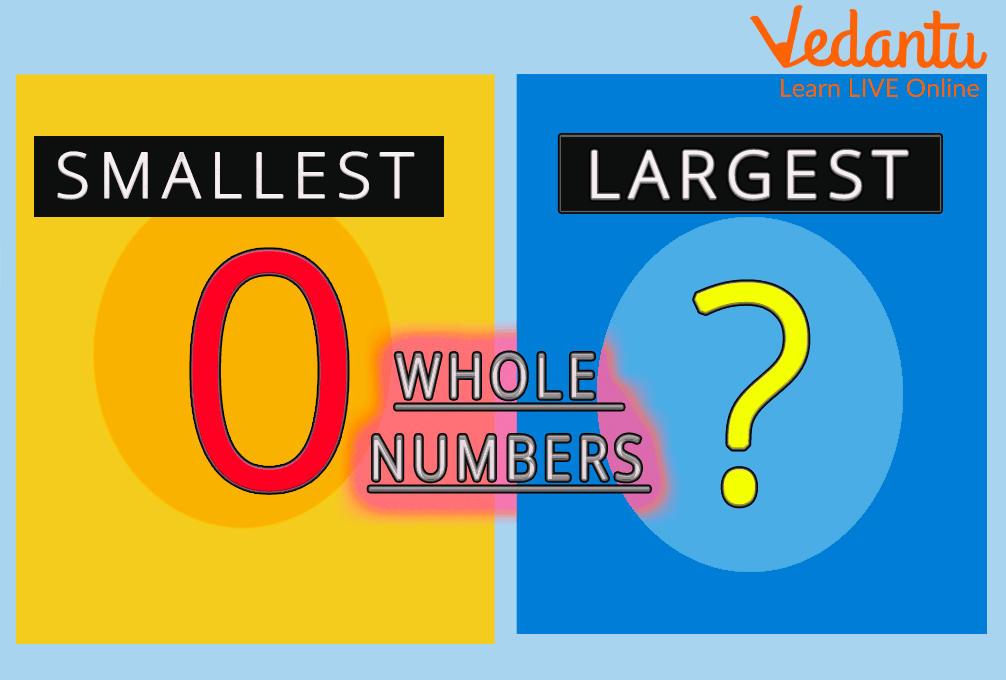Smallest Whole Numbers

## What are Whole Numbers?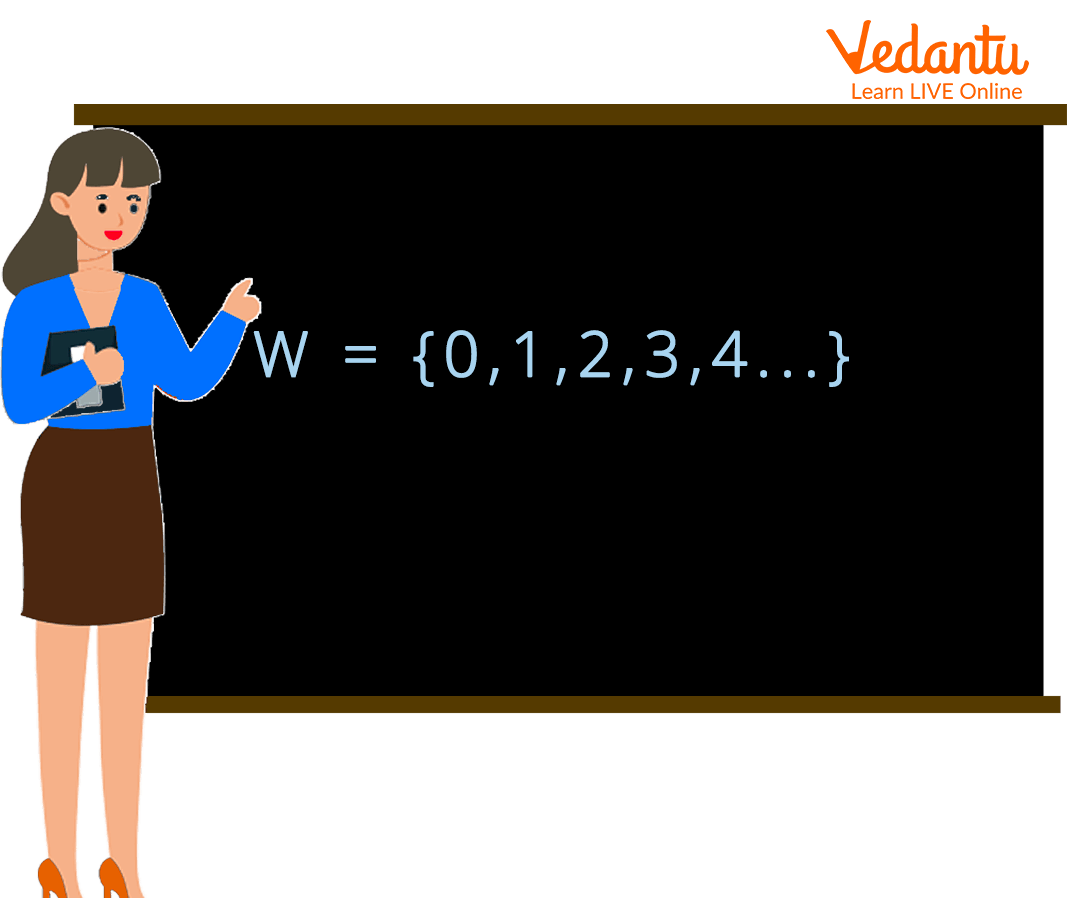Whole Numbers

The set of natural numbers and the number 0 are collectively referred to as whole numbers. The mathematical set of whole numbers is 0 through 9 and is represented by the letter W.

W = {0, 1, 2, 3, 4, …}

To better understand whole numbers, consider the following information:

• Whole numbers make up all natural numbers.

• There are only whole numbers when counting.

• Zero and all positive integers are whole numbers.

## Counting of Whole Numbers

Numbers that are used to count things and have some value are called counting numbers. Natural numbers in general are all counting numbers. Therefore, all whole numbers, including zero, are counting numbers.

## Whole Number Properties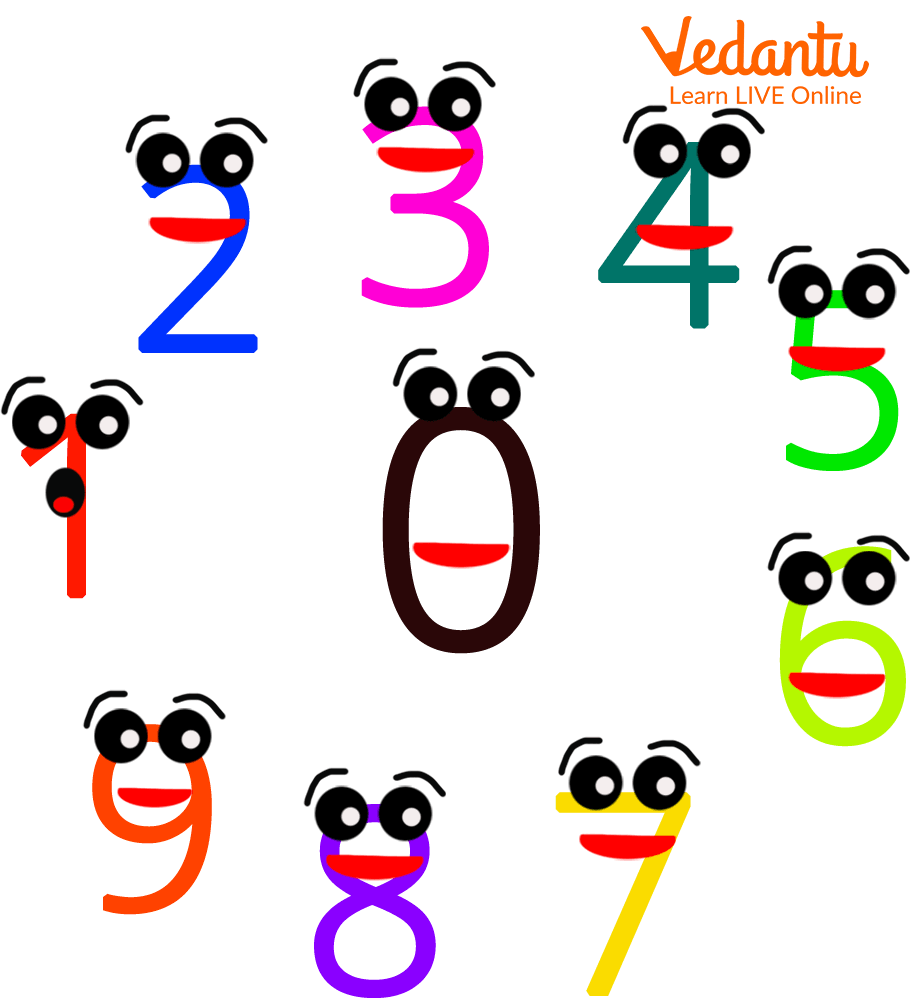Whole Number Properties

The product of two whole numbers is always a whole number that can be added to or multiplied by itself. There are three primary properties of whole numbers that are derived from the fundamental operations of addition, subtraction, multiplication, and division.

They are as follows:

1. Commutative Property: Even if the order of the numbers is reversed, the sum and product of whole numbers are the same.

$a+b=b+a$

$a \times b=b \times a$

For addition and multiplication, the commutativity property holds true.

1. Associative Property: The associative property states that the sum or product is unaffected by the order in which the whole numbers are added together or multiplied.

$a+(b+c)=(a+b)+c$

$a \times(b \times c)=(a \times b) \times c$

1. Distributive Property: The distributive property is the distribution of a whole number's multiplication over its sum or difference with other whole numbers. When the distributive property is applied, the problem is easier to solve.

$a \times(b+c)=(a \times b)+(a \times c)$

## Types of Whole Numbers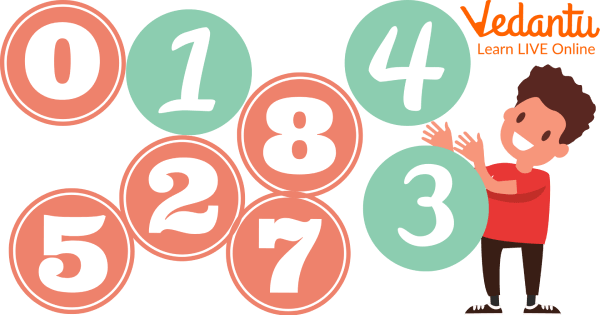Whole Numbers

Different names are given to different types of whole numbers.

• Natural Numbers

• Whole Numbers

• Integers

## Natural Numbers

A natural number is a whole number starting at 1 and going up:

1,2,3,4,5,6,7,8,9,10….

Counting numbers is another name for natural numbers. They are the numbers you would apply to count things. The smallest natural number is 1.

## Whole Numbers

Although any number without a fraction is a whole number, we also refer to the whole numbers starting at 0 as whole numbers:

0,1,2,3,4,5,6,7,8,9,….

## Integers

An integer is a whole number. An integer may be positive, negative, or zero:

-5,-4,-3,-2,-1,0,1,2,3,4,5,..

## Least Whole NumberLeast Whole Number

Whole numbers begin with 0, which is the smallest whole number (from the definition of whole numbers). Therefore, Zero is the smallest whole number.

Zero is used as a placeholder even though it has no value. Therefore, zero is a number that can neither be positive nor negative.

## Difference Between Natural and Whole Numbers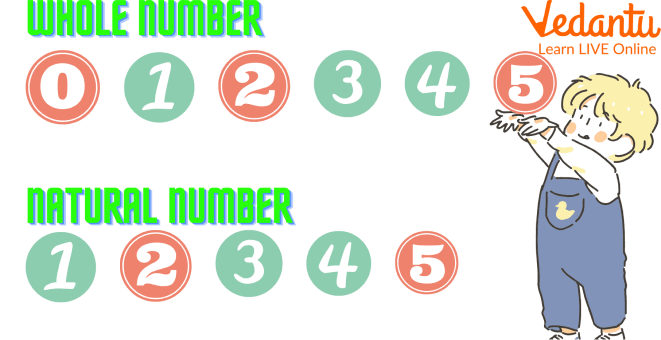Comparing Numbers

Following are the difference between natural and whole numbers:

 Natural Numbers Whole Numbers Natural numbers are generally used for counting objects or things. All-natural numbers and zero are considered whole numbers. The set of natural numbers is, N = {1,2,3,…}. W = 0, 1, 2, 3,... is the set of all whole numbers. The smallest natural number is 1. Zero is the least whole number. Represented by the letter ‘N’. Represented by the letter ‘W’.

We can easily assume from these differences that every whole number other than 0 is a natural number. We can assume that a subset of whole numbers is the set of natural numbers.

## Solved ExamplesLearning from Examples

Example 1: Solve 10 × (5 + 10) using the distributive property.

Ans: Distributive property of multiplication over the addition of whole numbers is:

x × (y + z) = (x × y) + (x × z)

10 × (5 + 10) = (10 × 5) + (10 × 10)

= 50 + 100

= 150

Therefore, 10 × (5 + 10) = 150

However, we can show several examples of whole numbers using the properties of the whole numbers.

Example 2: Make three different additions to the numbers. List the used property.

25 + 36 + 15

Ans: 25 + 36 + 15

• Step I: 25 + (36 + 15) = 25 + 51 = 76

• Step II: (25 + 36) + 15 = 61 + 15 = 76

• Step III: (25 + 15) + 36 = 40 + 36 = 76

Here, we have used associative property.

## Practice TimeLets Solve

1. Find the product of 6 × (40 + 2).

(Ans: 252)

2. How many whole numbers are there between 22 and 35?

(Ans: 12)

3. Write whole numbers between 12 and 25.

(Ans: 13, 14, 15, 16, 17, 18, 19, 20, 21, 22, 23, 24)

## Summary

In mathematics, any number that is not a fraction with a whole part is considered to be a whole number. Integers, rationals, and irrational numbers are all examples of whole numbers, while natural numbers are the subset of whole numbers which are either positive or zero. Whole numbers can be classified into two broad categories: natural and whole numbers. Natural numbers, also called counting numbers, are the set of integers starting at 1 and containing zero and every integer divisible by it. All other integers are called the non-zero natural number in contrast to "natural" ones.

## FAQs on The Smallest Whole Number - Zero

1. Can whole numbers be negative?

The whole numbers can't be negative, so no. Starting with 0, 1, 2, 3, and so forth, are the whole numbers. Although all whole numbers are regarded as being natural numbers, not all whole numbers are. Negative numbers are therefore not regarded as whole numbers.

2. What circumstances lead to the product of two whole numbers being zero?

One of the two must undoubtedly be zero if the product of two whole numbers is zero. As an illustration, 0 x 5 = 0 and 19 x 0 = 0

Both numbers could be zero if the product of two whole numbers is zero.

0 × 0 = 0

If one or both of the whole numbers are zero, the product of two whole numbers is also zero.

3. Which numbers do not fall under the whole number category?

Not all whole numbers fall within the range of 0 and infinity. Rational numbers, negative integers, and fractions are not whole numbers. Examples of non-whole numbers include -1, -5, 12, 9/4, pi, etc.

4. How many whole numbers are there between 23 and 36?

12 whole numbers are present between 23 and 36 and these are 24, 25, 26,27, 28, 29, 30, 31, 32, 33, 34, 35.